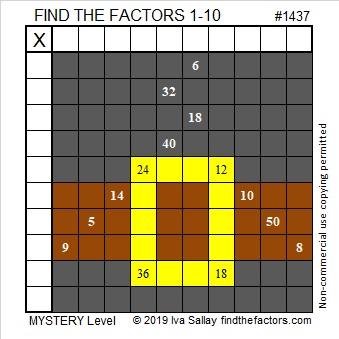# 1437 Belt Buckle Mystery

I have childhood memories of preparing for Thanksgiving at school by making pilgrim hats, pilgrim shirts, or pilgrim shoes out of construction paper. Each of those clothing items had a distinguished buckle. Why? That buckle’s popularity was a mystery to me until I read an article by Ken Jennings titled The Debunker: What Did Pilgrim Hats Really Look Like?

This mystery level puzzle has a buckle, too. Perhaps it can help debunk the idea that math is no fun while it helps you learn about factors or reinforces your memory of the multiplication table.Print the puzzles or type the solution in this excel file:  10 Factors 1432-1442

The puzzle number is 1437. Here are a few facts about that number:

• 1437 is a composite number.
• Prime factorization: 1437 = 3 × 479
• 1437 has no exponents greater than 1 in its prime factorization, so √1437 cannot be simplified.
• The exponents in the prime factorization are 1, and 1. Adding one to each exponent and multiplying we get (1 + 1)(1 + 1) = 2 × 2 = 4. Therefore 1437 has exactly 4 factors.
• The factors of 1437 are outlined with their factor pair partners in the graphic below.1437 is the difference of two squares in two different ways:
719² – 718² = 1437
241² – 238² = 1437